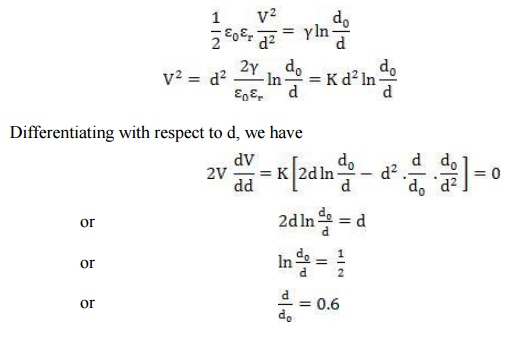Home | | High Voltage Engineering | Electromechanical Breakdown

# Electromechanical Breakdown

When a dielectric material is subjected to an electric field, charges of opposite nature are induced on the two opposite surfaces of the material .

ELECTROMECHANICAL BREAKDOWN

When a dielectric material is subjected to an electric field, charges of opposite nature are induced on the two opposite surfaces of the material and hence a force of attraction is developed and the specimen is subjected to electrostatic compressive forces and when these forces exceed the mechanical withstands strength of the material, the material collapses. If the initial thickness of the material is d0 and is compressed to a thickness d under the applied voltage V then the compressive stress developed due to electric field iswhere Îµr is the relative permittivity of the specimen. If Îł is the Youngâ€™s modulus, the mechanical compressive strength isEquating the two under equilibrium condition, we haveFor any real value of voltage V, the reduction in thickness of the specimen cannot be more than 40%. If the ratio V/d at this value of V is less than the intrinsic strength of the specimen, a further increase in V shall make the thickness unstable and the specimen collapses.

The highest apparent strength is then obtained by substituting d = 0.6 d0 in the above expressions.The above equation is approximate only as Îł depends upon the mechanical stress. The possibility of instability occurring for lower average field is ignored i.e., the effect of stress concentration at irregularities is not taken into account.

Study Material, Lecturing Notes, Assignment, Reference, Wiki description explanation, brief detail
High Voltage Engineering : Electrical breakdown is gases, solids& Liquids : Electromechanical Breakdown |